﻿ 网络交通流模型的高分辨率迎风格式

网络交通流模型的高分辨率迎风格式A High Resolution Upwind Scheme for Traffic Flow on Networks

Abstract: In this paper, the hyperbolic conservation law is used to describe the change law of the traffic flow density. Some typical examples of traffic flow problems are numerically simulated with a HPUS high-order bounded scheme that satisfies both CBC condition and TVD constraint. The results show that the scheme can effectively suppress non-physical oscillations near the discontinuities, and has good performance of numerical approximation.

1. 引言

2. 网络交通流模型

2.1. LWR模型

${\partial }_{t}\rho +{\partial }_{x}f\left(\rho \right)=0$

$\underset{i=1}{\overset{n}{\sum }}f\left({\rho }_{i}\left(t,{b}_{i}\right)\right)=\underset{j=n+1}{\overset{n+m}{\sum }}f\left({\rho }_{j}\left(t,{a}_{j}\right)\right)$

$A=\left(\begin{array}{cccc}{\alpha }_{n+1,1}& {\alpha }_{n+1,2}& \cdots & {\alpha }_{n+1,n}\\ {\alpha }_{n+2,1}& {\alpha }_{n+2,2}& \cdots & {\alpha }_{n+2,n}\\ ⋮& ⋮& \ddots & ⋮\\ {\alpha }_{n+m,1}& {\alpha }_{n+m,2}& \cdots & {\alpha }_{n+m,n}\end{array}\right)$

1) 矩阵A每一列的百分比相加和为1，即：

$\underset{j=n+1}{\overset{n+m}{\sum }}{\alpha }_{ji}=1,\text{\hspace{0.17em}}\left(j=n+1,\cdots ,n+m;\text{\hspace{0.17em}}i=1,2,\cdots ,n\right)$

2) 当满足条件1时，流通量函数 $f\left(\rho \right)$ 的最大值在交叉点处取到。若入路数比出路数多时，即当 $n>m$ 时，此结论不成立；

3) 当入路数比出路数多时，即当 $n>m$ 时。现假设交叉点入口处有a，b两条路，出口处只有c一条路，从a，b两条路驶入的车不一定都能从c驶出，可能有一部分会滞留在交叉口J处，故在出路c上要设定一个最大输出量Q，设 $q\left(0\le q\le 1\right)$ 为路径参数，记从入路a进来的车流量为 $q\cdot Q$，从入路b进来的车流量为 $\left(1-q\right)\cdot Q$

${\gamma }_{i}^{\mathrm{max}}=\left\{\begin{array}{l}f\left({\rho }_{i,0}\right),\text{\hspace{0.17em}}\text{\hspace{0.17em}}\text{\hspace{0.17em}}{\rho }_{i,0}\in \left[0,\sigma \right]\\ f\left(\sigma \right),\text{\hspace{0.17em}}\text{\hspace{0.17em}}\text{\hspace{0.17em}}\text{\hspace{0.17em}}\text{\hspace{0.17em}}{\rho }_{i,0}\in \left[\sigma ,{\rho }_{\mathrm{max}}\right]\end{array}\text{\hspace{0.17em}}\text{\hspace{0.17em}}\text{\hspace{0.17em}}i=1,\cdots ,n$

${\gamma }_{j}^{\mathrm{max}}=\left\{\begin{array}{l}f\left({\rho }_{j,0}\right),\text{\hspace{0.17em}}\text{\hspace{0.17em}}\text{ }\text{ }{\rho }_{j,0}\in \left[0,\sigma \right]\\ f\left(\sigma \right),\text{\hspace{0.17em}}\text{\hspace{0.17em}}\text{\hspace{0.17em}}\text{\hspace{0.17em}}\text{\hspace{0.17em}}{\rho }_{j,0}\in \left[\sigma ,{\rho }_{\mathrm{max}}\right]\end{array}\text{\hspace{0.17em}}\text{\hspace{0.17em}}\text{\hspace{0.17em}}j=n+1,\cdots ,n+m$

2.2. 交叉点处流通量

2.2.1. 一条入路a和一条出路b

${\stackrel{^}{\gamma }}_{a}={\stackrel{^}{\gamma }}_{b}=\stackrel{^}{\gamma },\text{\hspace{0.17em}}\text{\hspace{0.17em}}\text{\hspace{0.17em}}\stackrel{^}{\gamma }=\mathrm{min}\left\{{\gamma }_{a}^{\mathrm{max}},{\gamma }_{b}^{\mathrm{max}}\right\}$

${\stackrel{^}{f}}_{{N}_{a}+\frac{1}{2}}^{a}={\stackrel{^}{\gamma }}_{a},\text{\hspace{0.17em}}\text{\hspace{0.17em}}\text{\hspace{0.17em}}{\stackrel{^}{f}}_{\frac{1}{2}}^{b}={\stackrel{^}{\gamma }}_{b}$

2.2.2. 两条入路a、b和一条出路c

Case1：两条入路在J处的最大流通量之和小于出入在J处的最大流通量，即当 ${\stackrel{^}{\gamma }}_{a}^{\mathrm{max}}+{\stackrel{^}{\gamma }}_{b}^{\mathrm{max}}\le {\stackrel{^}{\gamma }}_{c}^{\mathrm{max}}$ 时，两条入路的车流均可顺利通过交叉点到达出路c，则记

${\stackrel{^}{\gamma }}_{a}={\gamma }_{a}^{\mathrm{max}},\text{\hspace{0.17em}}\text{\hspace{0.17em}}\text{\hspace{0.17em}}{\stackrel{^}{\gamma }}_{b}={\gamma }_{b}^{\mathrm{max}},\text{\hspace{0.17em}}\text{\hspace{0.17em}}\text{\hspace{0.17em}}{\stackrel{^}{\gamma }}_{c}={\stackrel{^}{\gamma }}_{a}+{\stackrel{^}{\gamma }}_{b}$

Case2：两条入路在J处的最大流通量之和大于出入在J处的最大流通量，即当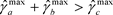时，两条入路的车流量之和超出出路c可承受车流量，故两条入路的车流需按2.1中条件3中所给出的比例进入c车道。现取路径参数为 $q\left(0\le q\le 1\right)$，此时c车道上的最大流通量为 ${\gamma }_{c}^{\mathrm{max}}$，则由路a驶入路c的车流量为 $q\cdot {\gamma }_{c}^{\mathrm{max}}$，由路b驶入路c的车流量为 $\left(1-q\right)\cdot {\gamma }_{c}^{\mathrm{max}}$，则交叉点J处三条路的实际流通量应满足以下三种情况：

1) 当 ${\gamma }_{a}^{\mathrm{max}}\ge q{\gamma }_{c}^{\mathrm{max}},{\gamma }_{b}^{\mathrm{max}}\ge \left(1-q\right){\gamma }_{c}^{\mathrm{max}}$ 时，则有

${\stackrel{^}{\gamma }}_{a}=q{\gamma }_{c}^{\mathrm{max}},\text{\hspace{0.17em}}\text{\hspace{0.17em}}{\stackrel{^}{\gamma }}_{b}=\left(1-q\right){\gamma }_{c}^{\mathrm{max}},\text{\hspace{0.17em}}\text{\hspace{0.17em}}{\stackrel{^}{\gamma }}_{c}={\gamma }_{c}^{\mathrm{max}}$

2) 当 ${\gamma }_{a}^{\mathrm{max}} 时，则有

${\stackrel{^}{\gamma }}_{a}={\gamma }_{a}^{\mathrm{max}},\text{\hspace{0.17em}}\text{\hspace{0.17em}}{\stackrel{^}{\gamma }}_{b}={\gamma }_{c}^{\mathrm{max}}-{\gamma }_{a}^{\mathrm{max}},\text{\hspace{0.17em}}\text{\hspace{0.17em}}{\stackrel{^}{\gamma }}_{c}={\gamma }_{c}^{\mathrm{max}}$

3) 当 ${\gamma }_{a}^{\mathrm{max}}\ge q{\gamma }_{c}^{\mathrm{max}},{\gamma }_{b}^{\mathrm{max}}<\left(1-q\right){\gamma }_{c}^{\mathrm{max}}$ 时，则有

${\stackrel{^}{\gamma }}_{a}={\gamma }_{c}^{\mathrm{max}}-{\gamma }_{b}^{\mathrm{max}},\text{\hspace{0.17em}}\text{\hspace{0.17em}}{\stackrel{^}{\gamma }}_{b}={\gamma }_{b}^{\mathrm{max}},\text{\hspace{0.17em}}\text{\hspace{0.17em}}{\stackrel{^}{\gamma }}_{c}={\gamma }_{c}^{\mathrm{max}}$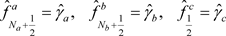3. HPUS格式

${\partial }_{t}\rho +{\partial }_{x}f\left(\rho \right)=0$ (1)

3.1. 空间离散

$\frac{\text{d}{\stackrel{¯}{\rho }}_{j}\left(t\right)}{\text{d}t}=-\frac{1}{\Delta x}\left({\stackrel{^}{f}}_{j+\frac{1}{2}}-{\stackrel{^}{f}}_{j-\frac{1}{2}}\right)$

$\begin{array}{l}{\stackrel{^}{f}}_{j+\frac{1}{2}}=\frac{1}{2}\left[\stackrel{^}{f}\left({\rho }_{j+\frac{1}{2}}^{+}\right)+\stackrel{^}{f}\left({\rho }_{j+\frac{1}{2}}^{-}\right)-\alpha \left({\rho }_{j+\frac{1}{2}}^{-}-{\rho }_{j+\frac{1}{2}}^{+}\right)\right]\\ {\stackrel{^}{f}}_{j-\frac{1}{2}}=\frac{1}{2}\left[\stackrel{^}{f}\left({\rho }_{j-\frac{1}{2}}^{+}\right)+\stackrel{^}{f}\left({\rho }_{j-\frac{1}{2}}^{-}\right)-\alpha \left({\rho }_{j-\frac{1}{2}}^{-}-{\rho }_{j-\frac{1}{2}}^{+}\right)\right]\end{array}$

3.2. 对流有界性Figure 1. Three neighboring mesh points and the mesh face

${\varphi }_{f}={\varphi }_{C}+\left[\frac{1+\kappa }{4}\left({\varphi }_{D}-{\varphi }_{C}\right)+\frac{1-\kappa }{4}\left({\varphi }_{C}-{\varphi }_{U}\right)\right]$

$\stackrel{^}{\varphi }=\frac{\varphi -{\varphi }_{U}}{{\varphi }_{D}-{\varphi }_{U}}$

${\stackrel{^}{\varphi }}_{f}=\left(1-\frac{1}{2}k\right){\stackrel{^}{\varphi }}_{c}+\frac{1}{4}\left(1+k\right)$Table 1. System resulting data of standard experiment

$\left\{\begin{array}{l}\frac{3}{2}{\stackrel{^}{\varphi }}_{c}\le f\left({\stackrel{^}{\varphi }}_{c}\right)<\frac{1}{2}\left({\stackrel{^}{\varphi }}_{c}+1\right),\text{\hspace{0.17em}}\text{\hspace{0.17em}}\text{\hspace{0.17em}}\text{\hspace{0.17em}}\text{\hspace{0.17em}}\text{\hspace{0.17em}}\text{\hspace{0.17em}}\text{\hspace{0.17em}}\text{\hspace{0.17em}}\text{\hspace{0.17em}}\text{\hspace{0.17em}}\text{\hspace{0.17em}}\text{\hspace{0.17em}}\text{\hspace{0.17em}}\text{ }\text{ }\text{\hspace{0.17em}}\text{\hspace{0.17em}}\text{\hspace{0.17em}}\text{\hspace{0.17em}}\text{\hspace{0.17em}}\text{\hspace{0.17em}}0<{\stackrel{^}{\varphi }}_{c}<\frac{1}{2}\\ \frac{1}{2}\left({\stackrel{^}{\varphi }}_{c}+1\right)\le f\left({\stackrel{^}{\varphi }}_{c}\right)\le \frac{3}{2}{\stackrel{^}{\varphi }}_{c},f\left({\stackrel{^}{\varphi }}_{c}\right)\le 1,\text{\hspace{0.17em}}\text{\hspace{0.17em}}\text{\hspace{0.17em}}\text{\hspace{0.17em}}\text{\hspace{0.17em}}\text{\hspace{0.17em}}\text{\hspace{0.17em}}\frac{1}{2}\le {\stackrel{^}{\varphi }}_{c}<1\\ {\stackrel{^}{\varphi }}_{f}={\stackrel{^}{\varphi }}_{c},\text{\hspace{0.17em}}\text{\hspace{0.17em}}\text{\hspace{0.17em}}\text{\hspace{0.17em}}\text{\hspace{0.17em}}\text{\hspace{0.17em}}\text{\hspace{0.17em}}\text{\hspace{0.17em}}\text{\hspace{0.17em}}\text{\hspace{0.17em}}\text{\hspace{0.17em}}\text{\hspace{0.17em}}\text{\hspace{0.17em}}\text{\hspace{0.17em}}\text{\hspace{0.17em}}\text{\hspace{0.17em}}\text{\hspace{0.17em}}\text{\hspace{0.17em}}\text{\hspace{0.17em}}\text{\hspace{0.17em}}\text{\hspace{0.17em}}\text{\hspace{0.17em}}\text{\hspace{0.17em}}\text{\hspace{0.17em}}\text{\hspace{0.17em}}\text{\hspace{0.17em}}\text{\hspace{0.17em}}\text{\hspace{0.17em}}\text{\hspace{0.17em}}\text{\hspace{0.17em}}\text{\hspace{0.17em}}\text{\hspace{0.17em}}\text{\hspace{0.17em}}\text{\hspace{0.17em}}\text{\hspace{0.17em}}\text{\hspace{0.17em}}\text{\hspace{0.17em}}\text{\hspace{0.17em}}\text{\hspace{0.17em}}\text{\hspace{0.17em}}\text{\hspace{0.17em}}\text{ }\text{\hspace{0.17em}}\text{\hspace{0.17em}}\text{\hspace{0.17em}}\text{\hspace{0.17em}}\text{\hspace{0.17em}}{\stackrel{^}{\varphi }}_{c}\ge 1\\ {\stackrel{^}{\varphi }}_{f}={\stackrel{^}{\varphi }}_{c},\text{\hspace{0.17em}}\text{\hspace{0.17em}}\text{\hspace{0.17em}}\text{\hspace{0.17em}}\text{\hspace{0.17em}}\text{\hspace{0.17em}}\text{\hspace{0.17em}}\text{\hspace{0.17em}}\text{\hspace{0.17em}}\text{\hspace{0.17em}}\text{\hspace{0.17em}}\text{\hspace{0.17em}}\text{\hspace{0.17em}}\text{\hspace{0.17em}}\text{\hspace{0.17em}}\text{\hspace{0.17em}}\text{\hspace{0.17em}}\text{\hspace{0.17em}}\text{\hspace{0.17em}}\text{\hspace{0.17em}}\text{\hspace{0.17em}}\text{\hspace{0.17em}}\text{\hspace{0.17em}}\text{\hspace{0.17em}}\text{\hspace{0.17em}}\text{\hspace{0.17em}}\text{\hspace{0.17em}}\text{\hspace{0.17em}}\text{\hspace{0.17em}}\text{\hspace{0.17em}}\text{\hspace{0.17em}}\text{\hspace{0.17em}}\text{\hspace{0.17em}}\text{\hspace{0.17em}}\text{\hspace{0.17em}}\text{\hspace{0.17em}}\text{\hspace{0.17em}}\text{\hspace{0.17em}}\text{\hspace{0.17em}}\text{\hspace{0.17em}}\text{\hspace{0.17em}}\text{\hspace{0.17em}}\text{\hspace{0.17em}}\text{\hspace{0.17em}}\text{\hspace{0.17em}}\text{\hspace{0.17em}}{\stackrel{^}{\varphi }}_{c}\le 0\end{array}$

$\left\{\begin{array}{l}{\stackrel{^}{\varphi }}_{f}\le 1,\text{\hspace{0.17em}}{\stackrel{^}{\varphi }}_{f}\in \left[{\stackrel{^}{\varphi }}_{c},2{\stackrel{^}{\varphi }}_{c}\right],\text{\hspace{0.17em}}\text{\hspace{0.17em}}\text{\hspace{0.17em}}\text{\hspace{0.17em}}0<{\stackrel{^}{\varphi }}_{c}<1\\ {\stackrel{^}{\varphi }}_{f}={\stackrel{^}{\varphi }}_{c},\text{\hspace{0.17em}}\text{\hspace{0.17em}}\text{\hspace{0.17em}}\text{\hspace{0.17em}}\text{\hspace{0.17em}}\text{\hspace{0.17em}}\text{\hspace{0.17em}}\text{\hspace{0.17em}}\text{\hspace{0.17em}}\text{\hspace{0.17em}}\text{\hspace{0.17em}}\text{\hspace{0.17em}}\text{\hspace{0.17em}}\text{\hspace{0.17em}}\text{\hspace{0.17em}}\text{\hspace{0.17em}}\text{\hspace{0.17em}}\text{\hspace{0.17em}}\text{\hspace{0.17em}}\text{\hspace{0.17em}}\text{\hspace{0.17em}}\text{\hspace{0.17em}}\text{\hspace{0.17em}}\text{\hspace{0.17em}}{\stackrel{^}{\varphi }}_{c}\le 0\\ {\stackrel{^}{\varphi }}_{f}={\stackrel{^}{\varphi }}_{c},\text{\hspace{0.17em}}\text{\hspace{0.17em}}\text{\hspace{0.17em}}\text{\hspace{0.17em}}\text{\hspace{0.17em}}\text{\hspace{0.17em}}\text{\hspace{0.17em}}\text{\hspace{0.17em}}\text{\hspace{0.17em}}\text{\hspace{0.17em}}\text{\hspace{0.17em}}\text{\hspace{0.17em}}\text{\hspace{0.17em}}\text{\hspace{0.17em}}\text{\hspace{0.17em}}\text{\hspace{0.17em}}\text{\hspace{0.17em}}\text{\hspace{0.17em}}\text{\hspace{0.17em}}\text{\hspace{0.17em}}\text{\hspace{0.17em}}\text{\hspace{0.17em}}\text{\hspace{0.17em}}\text{\hspace{0.17em}}{\stackrel{^}{\varphi }}_{c}\ge 1\end{array}$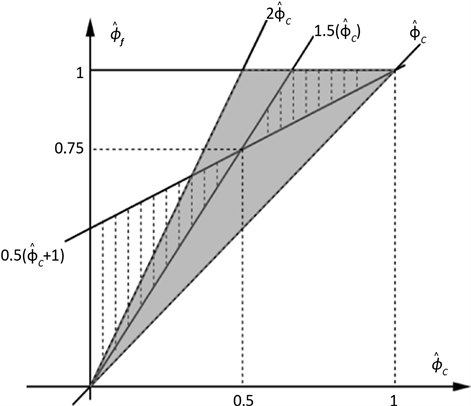Figure 2. The regions of the TVD (shaded) and BAIR (hatched)

3.3. 对流项离散

1) 若对流格式的NV线过点 $\left(\frac{1}{2},\frac{3}{4}\right)$，则该式具有二阶局部截断误差。

2) 对流格式的NV线需满足 $f\left(\frac{1}{2}\right)=\frac{3}{4},{f}^{\prime }\left(\frac{1}{2}\right)=1-\frac{1}{2}k$

3) 为使对流格式同时满足BAIR准则和TVD约束条件，格式的图像应落在图中阴影部分且 ${\stackrel{^}{\varphi }}_{c}\in \left[0,1\right]$

${\stackrel{^}{\varphi }}_{f}=\left\{\begin{array}{l}{\stackrel{^}{\varphi }}_{c}\left(-4{\stackrel{^}{\varphi }}_{c}^{4}+10{\stackrel{^}{\varphi }}_{c}^{3}-8{\stackrel{^}{\varphi }}_{c}^{2}+{\stackrel{^}{\varphi }}_{c}+2\right),\text{\hspace{0.17em}}\text{\hspace{0.17em}}\text{\hspace{0.17em}}0<{\stackrel{^}{\varphi }}_{c}<1\\ {\stackrel{^}{\varphi }}_{c},\text{\hspace{0.17em}}\text{\hspace{0.17em}}\text{\hspace{0.17em}}\text{\hspace{0.17em}}\text{\hspace{0.17em}}\text{\hspace{0.17em}}\text{\hspace{0.17em}}\text{\hspace{0.17em}}\text{\hspace{0.17em}}\text{\hspace{0.17em}}\text{\hspace{0.17em}}\text{\hspace{0.17em}}\text{\hspace{0.17em}}\text{\hspace{0.17em}}\text{\hspace{0.17em}}\text{\hspace{0.17em}}\text{\hspace{0.17em}}\text{\hspace{0.17em}}\text{\hspace{0.17em}}\text{\hspace{0.17em}}\text{\hspace{0.17em}}\text{\hspace{0.17em}}\text{\hspace{0.17em}}\text{\hspace{0.17em}}\text{\hspace{0.17em}}\text{\hspace{0.17em}}\text{\hspace{0.17em}}\text{\hspace{0.17em}}\text{\hspace{0.17em}}\text{\hspace{0.17em}}\text{\hspace{0.17em}}\text{\hspace{0.17em}}\text{\hspace{0.17em}}\text{\hspace{0.17em}}\text{\hspace{0.17em}}\text{\hspace{0.17em}}\text{\hspace{0.17em}}\text{\hspace{0.17em}}\text{\hspace{0.17em}}\text{\hspace{0.17em}}\text{\hspace{0.17em}}\text{\hspace{0.17em}}其他\end{array}$

3.4. 时间离散

$\left\{\begin{array}{l}{\rho }^{\left(1\right)}={\rho }^{n}+\Delta tL\left({\rho }^{n}\right)\\ {\rho }^{\left(2\right)}=\frac{3}{4}{\rho }^{n}+\frac{1}{4}\left[{\rho }^{\left(1\right)}+\Delta tL\left({\rho }^{\left(1\right)}\right)\right]\\ {\rho }^{n+1}=\frac{1}{3}{\rho }^{n}+\frac{2}{3}\left[{\rho }^{\left(2\right)}+\Delta tL\left({\rho }^{\left(2\right)}\right)\right]\end{array}$

4. 数值算例

4.1. Bottleneck问题

${f}_{1}\left(\rho \right)=\rho \left(1-\rho \right),\text{\hspace{0.17em}}\text{\hspace{0.17em}}\rho \in \left[0,1\right]$

${\sigma }_{1}=\frac{1}{2}$ 时，可取到入路a上的最大流通量： ${f}_{1}\left({\sigma }_{1}\right)=\underset{\rho \in \left[0,1\right]}{\mathrm{max}}{f}_{1}\left(\rho \right)=\frac{1}{4}$

${f}_{2}\left(\rho \right)=\rho \left(1-\frac{3}{2}\rho \right),\text{\hspace{0.17em}}\text{\hspace{0.17em}}\rho \in \left[0,\frac{2}{3}\right]$

${\sigma }_{2}=\frac{1}{3}$ 时，可取到出路b上的最大流通量： ${f}_{2}\left({\sigma }_{2}\right)=\underset{\rho \in \left[0,\frac{2}{3}\right]}{\mathrm{max}}{f}_{2}\left(\rho \right)=\frac{1}{6}$

$\begin{array}{l}\left\{\begin{array}{l}{\rho }_{1}\left(0,x\right)=0.66,\text{\hspace{0.17em}}\text{\hspace{0.17em}}\text{ }x\in \left[0,1\right]\\ {\rho }_{2}\left(0,x\right)=0.66,\text{\hspace{0.17em}}\text{\hspace{0.17em}}x\in \left[1,2\right]\end{array}\\ {\rho }_{1,b}\left(t,0\right)=0.25\end{array}$

$\begin{array}{l}\left\{\begin{array}{l}{\rho }_{1}\left(0,x\right)=0,\text{\hspace{0.17em}}\text{\hspace{0.17em}}\text{ }x\in \left[0,1\right]\\ {\rho }_{2}\left(0,x\right)=0,\text{\hspace{0.17em}}\text{\hspace{0.17em}}x\in \left[1,2\right]\end{array}\\ {\rho }_{1,b}\left(t\right)={\rho }_{1}\left(t,0\right)=0.4\end{array}$

$\begin{array}{l}\left\{\begin{array}{l}{\rho }_{1}\left(0,x\right)=0.4+0.2\mathrm{sin}\left(5\pi x\right),\text{\hspace{0.17em}}\text{\hspace{0.17em}}\text{\hspace{0.17em}}x\in \left[0,1\right]\\ {\rho }_{2}\left(0,x\right)=0.66,\text{\hspace{0.17em}}\text{\hspace{0.17em}}\text{\hspace{0.17em}}\text{\hspace{0.17em}}\text{\hspace{0.17em}}\text{\hspace{0.17em}}\text{\hspace{0.17em}}\text{\hspace{0.17em}}\text{\hspace{0.17em}}\text{\hspace{0.17em}}\text{\hspace{0.17em}}\text{\hspace{0.17em}}\text{\hspace{0.17em}}\text{\hspace{0.17em}}\text{\hspace{0.17em}}\text{\hspace{0.17em}}\text{\hspace{0.17em}}\text{\hspace{0.17em}}\text{\hspace{0.17em}}\text{\hspace{0.17em}}\text{ }\text{ }x\in \left[1,2\right]\end{array}\\ {\rho }_{1,b}\left(t\right)={\rho }_{1}\left(t,0\right)=0.25\end{array}$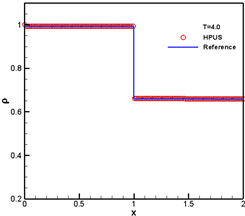Figure 3. Bottleneck problem with initial and boundary data—Case 1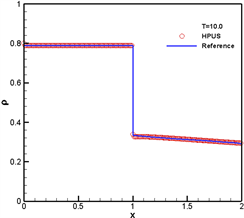Figure 4. Bottleneck problem with initial and boundary data—Case 2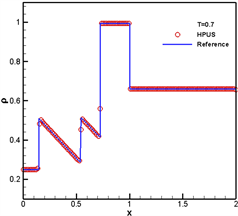Figure 5. Bottleneck problem with initial and boundary data—Case 3

4.2. 两条入路一条出路模型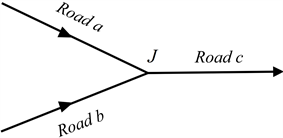Figure 6. A junction with two incoming and one outgoing roads

$\begin{array}{l}{\rho }_{1}\left(0,x\right)=\left\{\begin{array}{l}0.1+0.1{\mathrm{sin}}^{3}\left(5\pi x\right),\text{\hspace{0.17em}}\text{\hspace{0.17em}}\text{\hspace{0.17em}}\text{\hspace{0.17em}}x\in \left[0,0.2\right]\cup \left[0.4,0.6\right]\cup \left[0.8,1\right]\\ 0.2,\text{\hspace{0.17em}}\text{\hspace{0.17em}}\text{\hspace{0.17em}}\text{\hspace{0.17em}}\text{\hspace{0.17em}}\text{\hspace{0.17em}}\text{\hspace{0.17em}}\text{\hspace{0.17em}}\text{\hspace{0.17em}}\text{\hspace{0.17em}}\text{\hspace{0.17em}}\text{\hspace{0.17em}}\text{\hspace{0.17em}}\text{\hspace{0.17em}}\text{\hspace{0.17em}}\text{\hspace{0.17em}}\text{\hspace{0.17em}}\text{\hspace{0.17em}}\text{\hspace{0.17em}}\text{\hspace{0.17em}}\text{\hspace{0.17em}}\text{\hspace{0.17em}}\text{\hspace{0.17em}}\text{\hspace{0.17em}}\text{\hspace{0.17em}}\text{\hspace{0.17em}}\text{\hspace{0.17em}}\text{\hspace{0.17em}}\text{\hspace{0.17em}}\text{\hspace{0.17em}}\text{\hspace{0.17em}}\text{\hspace{0.17em}}\text{\hspace{0.17em}}\text{\hspace{0.17em}}\text{\hspace{0.17em}}\text{\hspace{0.17em}}\text{\hspace{0.17em}}\text{\hspace{0.17em}}\text{\hspace{0.17em}}\text{\hspace{0.17em}}\text{\hspace{0.17em}}\text{\hspace{0.17em}}\text{\hspace{0.17em}}其他\end{array}\\ {\rho }_{2}\left(0,x\right)=\left\{\begin{array}{l}0,\text{\hspace{0.17em}}\text{\hspace{0.17em}}\text{\hspace{0.17em}}\text{\hspace{0.17em}}\text{\hspace{0.17em}}\text{\hspace{0.17em}}\text{\hspace{0.17em}}\text{\hspace{0.17em}}\text{\hspace{0.17em}}\text{\hspace{0.17em}}\text{\hspace{0.17em}}\text{\hspace{0.17em}}\text{\hspace{0.17em}}\text{\hspace{0.17em}}\text{\hspace{0.17em}}\text{\hspace{0.17em}}\text{\hspace{0.17em}}\text{\hspace{0.17em}}\text{\hspace{0.17em}}\text{\hspace{0.17em}}\text{\hspace{0.17em}}\text{\hspace{0.17em}}\text{\hspace{0.17em}}\text{\hspace{0.17em}}\text{\hspace{0.17em}}\text{\hspace{0.17em}}\text{\hspace{0.17em}}x\in \left[0,0.2\right]\cup \left[0.4,0.6\right]\cup \left[0.8,1\right]\\ 0.1+0.1\mathrm{sin}\left(5\pi x\right),\text{\hspace{0.17em}}\text{\hspace{0.17em}}\text{\hspace{0.17em}}\text{\hspace{0.17em}}\text{\hspace{0.17em}}\text{\hspace{0.17em}}\text{\hspace{0.17em}}\text{\hspace{0.17em}}\text{\hspace{0.17em}}\text{\hspace{0.17em}}\text{\hspace{0.17em}}\text{\hspace{0.17em}}\text{\hspace{0.17em}}\text{\hspace{0.17em}}\text{\hspace{0.17em}}\text{\hspace{0.17em}}\text{\hspace{0.17em}}\text{\hspace{0.17em}}\text{\hspace{0.17em}}\text{\hspace{0.17em}}\text{\hspace{0.17em}}\text{\hspace{0.17em}}\text{\hspace{0.17em}}\text{\hspace{0.17em}}其他\end{array}\\ {\rho }_{3}\left(0,x\right)=0.1\end{array}$

$\begin{array}{l}{\rho }_{1,b}\left(t,0\right)=0.1\\ {\rho }_{2,b}\left(t,0\right)=0.1\end{array}$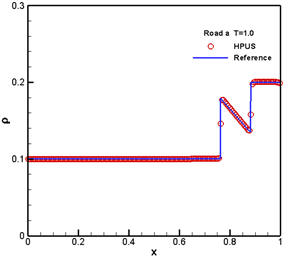Figure 7. Numerical results for Road a at T = 0.5, 1.0.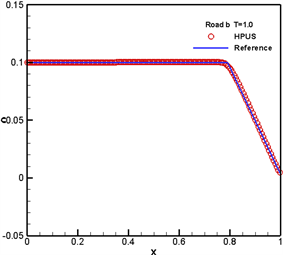Figure 8. Numerical results for Road b at T = 0.5, 1.0.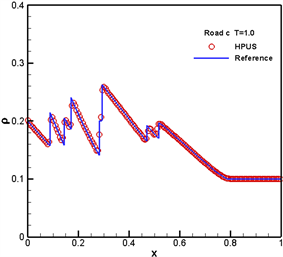Figure 9. Numerical results for Road c at T = 0.5, 1.0.

5. 结论

 Richards, P.I. (1956) Shock Waves on the Highway. Operations Research, 4, 42-51.
https://doi.org/10.1287/opre.4.1.42

 Pipes, L.A. (1953) An Operational Analysis of Traffic Dynamics. Journal of Applied Physics, 24, 274-281.
https://doi.org/10.1063/1.1721265

 Lighthill, M.J. and Whitham, G.B. (1955) On Kinematic Waves. II. A Theory of Traffic Flow on Long Crowded Roads, Proceedings of the Royal Society of London. Series A, 229, 317-345.
https://doi.org/10.1098/rspa.1955.0089

 Payne, H.J. (1973) Freeway Traffic Control and Surveillance Model. Journal of Transportation Engineering, 99, 767-783.

 Spalding, D.B. (1972) A Novel Finite Difference Formulation for Differential Expressions Involving Both First and Second Derivatives. International Journal for Numerical Methods in Engineering, 4, 551-559.
https://doi.org/10.1002/nme.1620040409

 Leonard, B.P. (1979) A Stable and Accurate Modeling Procedure Based on Quadratic Interpolation. Computer Methods in Applied Mechanics and Engineering, 19, 59-98.
https://doi.org/10.1016/0045-7825(79)90034-3

 Agarwal, R.K. (1981) A Third-Order-Accurate Upwind Scheme for Navier-Stokes Solutions at high Reynolds Num bers. 19th AIAA Aerospace Sciences Meeting, St. Louis, MO, 12-15 January 1981.
https://doi.org/10.2514/6.1981-112

 Leonard, B.P. (1988) Simple High-Accuracy Resolution Program for Convective Modeling of Discontinuities. International Journal for Numerical Methods in Fluids, 8, 1291-1318.
https://doi.org/10.1002/fld.1650081013

 Harten, A. (1983) High Resolution Schemes for Hyperbolic Conservation Law. Journal of Computational Physics, 49, 4357-4393.
https://doi.org/10.1016/0021-9991(83)90136-5

 Gao, W., Li, H. and Liu, Y. (2013) A High Resolution NV/TVD Hermite Polynomial Upwind Scheme for Convection- dominated Problems. Mathematical Methods in the Applied Sciences, 36, 1107-1122.
https://doi.org/10.1002/mma.2667

 Bretti, G., Natalini, R. and Piccoli, B. (2007) A Fluid-Dynamic Traffic Model on Road Networks. Archives of Computational Methods in Engineering, 14, 139-172.
https://doi.org/10.1007/s11831-007-9004-8

 Coclite, G.M., Garavello, M. and Piccoli, B. (2005) Traffic Flow on a Road Network. SIAM Journal on Mathematical Analysis, 36, 1862-1886.
https://doi.org/10.1137/S0036141004402683

 Bretti, G., Natalini, R. and Piccoli, B. (2006) Numerical Approximations of a Traffic Flow Model on Networks. Networks & Heterogeneous Media, 1, 57-84.
https://doi.org/10.3934/nhm.2006.1.57

 Canic, S., Piccoli, B., Qiu, J.-M. and Ren, T. (2015) Runge-Kutta Discontinuous Galerkin Method for Traffic Flow Model on Networks. Journal of Scientific Computing, 63, 233-255.
https://doi.org/10.1007/s10915-014-9896-z

 Garavello, M. and Piccoli, B. (2006) Traffic Flow on Networks, Volume 1. American Institute of Mathematical Sciences, Springfield, MO.

 Shi, Y.F. and Guo, Y. (2016) A Maximum-Principle-Satisfying Finite Volume Compact-WENO Scheme for Traffic Flow Model on Networks. Applied Numerical Mathematics, 108, 21-36.
https://doi.org/10.1016/j.apnum.2016.05.001

 Lax, P.D. and Wendroff, B. (1960) Systems of Conservations Laws. Communications on Pure and Applied Mathematics, 13, 217-237.
https://doi.org/10.1002/cpa.3160130205

 Wei, J.J., Yu, B., Tao, W.Q., Kawaguchi, Y. and Wang, H.S. (2003) A New High-Order-Accurate and Bounded Sche- me for Incompressible Flow. Numerical Heat Transfer, Part B: Fundamentals, 43, 19-41.
https://doi.org/10.1080/713836153

 Hou, P.L., Tao, W.Q. and Yu, M.Z. (2003) Refinement of the Convective Boundedness Criterion of Gaskell and Lau. Engineering Computations, 20, 1023-1043.
https://doi.org/10.1108/02644400310503008

 Zijlema, M. and Wesseling, P. (1995) Higher Order Flux-Limiting Methods for Steady-State, Multidimensional, Convection-Dominated Flow. Report 1995-131, TU Delft.

 Jiang, G.-S. and Shu, C.-W. (1996) Efficient Implementation of Weighted ENO Schemes. Journal of Computational Physics, 126, 202-228.
https://doi.org/10.1006/jcph.1996.0130

Top